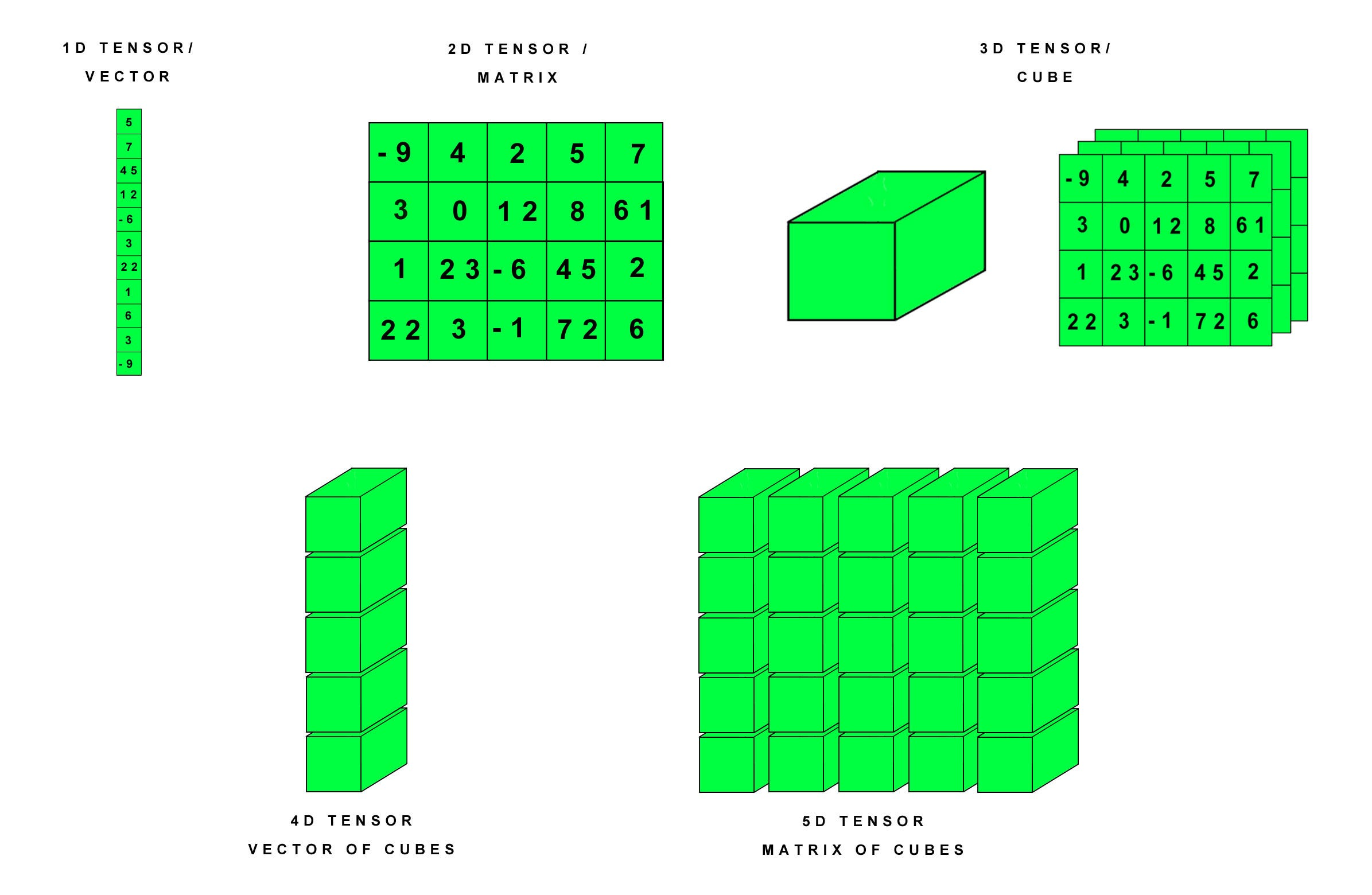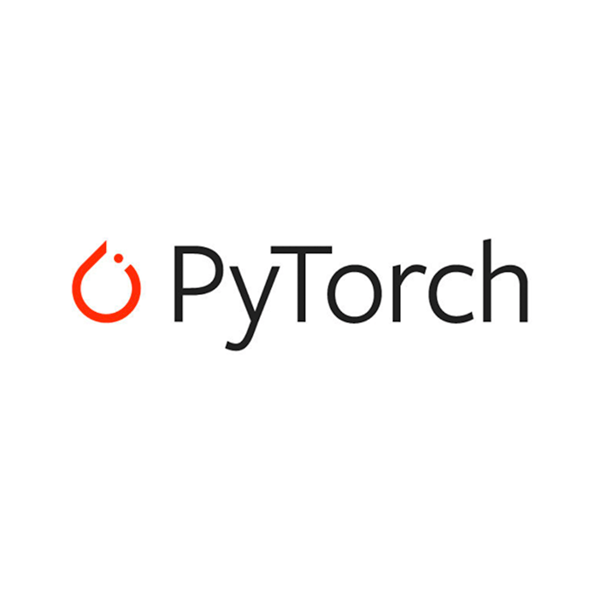1595580780

# Tensor operations in PyTorch

PyTorch is a scientific package based on Python, which is used to perform advanced operations using a special datatype known as Tensor. A Tensor is a number, vector, matrix, or multi-dimensional array with regular shape and numbers of the same datatype. PyTorch is an alternative to the NumPy package, which can additionally be used with the power of GPUs. It is also used as a framework for conducting research in deep learning.Understanding tensors

The 5 operations are:

• expand()
• permute()
• tolist()
• narrow()
• where()

# 1. expand()

The existing tensor is expanded to new dimensions along the dimension with value 1. The tensor can be expanded along any one dimension or multiple dimensions at once. If you do not want to expand the tensor along a particular dimension, you can set its parametric value to -1.

Note: only singleton dimension can be expanded

In this example, the tensor has original dimensions as [1,2,3]. It is expanded to dimensions [2,2,3].

This function can be used to expand existing tensors along singleton dimensions. It only returns a new view and does not allocate new memory. Therefore, it can be used to study how the tensor behaves at larger dimensions.

# 2. permute()

This function returns a view of the tensor with the order of dimensions of original tensor changed according to our choice. For example, if the original dimensions are [1,2,3], we can change it to [3,2,1]. The function takes the required order of dimensions as its parameters.

#deep-learning #tensor #operations #jovian #pytorch #deep learning

## Buddha Community1595580780

## Tensor operations in PyTorch

PyTorch is a scientific package based on Python, which is used to perform advanced operations using a special datatype known as Tensor. A Tensor is a number, vector, matrix, or multi-dimensional array with regular shape and numbers of the same datatype. PyTorch is an alternative to the NumPy package, which can additionally be used with the power of GPUs. It is also used as a framework for conducting research in deep learning.Understanding tensors

The 5 operations are:

• expand()
• permute()
• tolist()
• narrow()
• where()

# 1. expand()

The existing tensor is expanded to new dimensions along the dimension with value 1. The tensor can be expanded along any one dimension or multiple dimensions at once. If you do not want to expand the tensor along a particular dimension, you can set its parametric value to -1.

Note: only singleton dimension can be expanded

In this example, the tensor has original dimensions as [1,2,3]. It is expanded to dimensions [2,2,3].

This function can be used to expand existing tensors along singleton dimensions. It only returns a new view and does not allocate new memory. Therefore, it can be used to study how the tensor behaves at larger dimensions.

# 2. permute()

This function returns a view of the tensor with the order of dimensions of original tensor changed according to our choice. For example, if the original dimensions are [1,2,3], we can change it to [3,2,1]. The function takes the required order of dimensions as its parameters.

#deep-learning #tensor #operations #jovian #pytorch #deep learning1619565060

## Ternary operator in Python?

1. Ternary Operator in Python

What is a ternary operator: The ternary operator is a conditional expression that means this is a comparison operator and results come on a true or false condition and it is the shortest way to writing an if-else statement. It is a condition in a single line replacing the multiline if-else code.

syntax : condition ? value_if_true : value_if_false

condition: A boolean expression evaluates true or false

value_if_true: a value to be assigned if the expression is evaluated to true.

value_if_false: A value to be assigned if the expression is evaluated to false.

How to use ternary operator in python here are some examples of Python ternary operator if-else.

Brief description of examples we have to take two variables a and b. The value of a is 10 and b is 20. find the minimum number using a ternary operator with one line of code. ( **min = a if a < b else b ) **. if a less than b then print a otherwise print b and second examples are the same as first and the third example is check number is even or odd.

#python #python ternary operator #ternary operator #ternary operator in if-else #ternary operator in python #ternary operator with dict #ternary operator with lambda1591344961

Getting Started with PyTorch
PyTorch is a relatively new and robust deep learning framework, having a dynamic computation graph and supports flexibility. It was designed primarily by Soumith Chinatala, Facebook AI Research.

#tensor #pytorch #machine-learning #programming1617738420

## Unformatted input/output operations In C++

In this article, we will discuss the unformatted Input/Output operations In C++. Using objects cin and cout for the input and the output of data of various types is possible because of overloading of operator >> and << to recognize all the basic C++ types. The operator >> is overloaded in the istream class and operator << is overloaded in the ostream class.

The general format for reading data from the keyboard:

cin >> var1 >> var2 >> …. >> var_n;

• Here, var1var2, ……, varn are the variable names that are declared already.
• The input data must be separated by white space characters and the data type of user input must be similar to the data types of the variables which are declared in the program.
• The operator >> reads the data character by character and assigns it to the indicated location.
• Reading of variables terminates when white space occurs or character type occurs that does not match the destination type.1595575740

## Introduction to Pytorch and Tensors

I’ve taken up the 6-week course by Jovian.ml on “Deep Learning with PyTorch: Zero to GANs”taught by Aakash N S. In this article (and the following ones), I will share what I’ve learned during the course.

## Intro to PyTorch

Pytorch is an open-source machine learning and deep learning framework developed by Facebook. It is created to process large-scale image analysis, including object detection, recognition and classification and several other tasks. It is written in Python and C++ language. It can be used with other frameworks like Keras etc., to implement complex algorithms.

## Tensors

PyTorch is built on tensors. A PyTorch tensor is an n-dimensional array, similar to NumPy arrays.

## Installing PyTorch

Open the Anaconda terminal, type and run the following command:

conda install pytorch torchvision cudatoolkit=10.2 -c pytorch

## Using Pytorch

Start by importing the PyTorch library into your Jupyter Notebook. I’m importing it as tr (too lazy to type the whole thing). You can just import it as **torch **if you wish.

#tensor #pytorch #deep-learning #machine-learning #framework